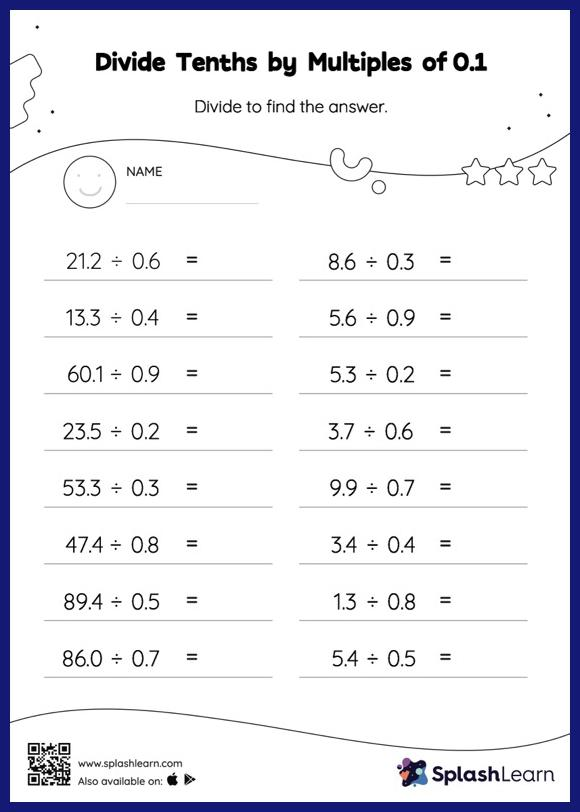# Divide Tenths by Multiples of 0.1 with Remainder: Horizontal Division Worksheet

Home > Divide Tenths by Multiples of 0.1 with Remainder: Horizontal DivisionThis worksheet strengthens students' accuracy and strategic flexibility while they divide tenths by multiples of 0.1 With remainder. Students first divide as though there is no decimal when dividing two decimals. They then add a decimal point at the correct location in the quotient depending on the number of decimal digits in each number. This divide tenths by multiples of 0.1 With remainder worksheet gives students a thorough practice of this concept.